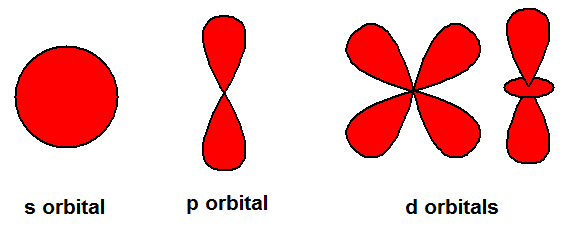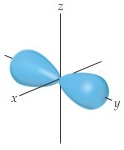# Problem: You may want to reference (Pages 232 - 235) Section 6.6 while completing this problem.The contour representation of one of the orbitals for the n = 3 shell of a hydrogen atom is shown in the figure below.In which of the following ways would you modify this sketch if the value of the magnetic quantum number, m𝓁, were to change?(i) It would be drawn larger(ii) The number of lobes would change(iii) The lobes of the orbital would point in a different direction(iv) There would be no change in the sketch

🤓 Based on our data, we think this question is relevant for Professor Atwood's class at UB.

###### FREE Expert Solution

Recall the different shapes of the s, p, and d orbitals.The m values (for each ℓ) represent an orbital for the subshell:

s subshell → 1 orbital
p subshell → 3 orbitals
d subshell → 5 orbitals

Possible set of quantum #'s for n = 3:

• ℓ = 0 to n - 1###### Problem Details

You may want to reference (Pages 232 - 235) Section 6.6 while completing this problem.

The contour representation of one of the orbitals for the n = 3 shell of a hydrogen atom is shown in the figure below.In which of the following ways would you modify this sketch if the value of the magnetic quantum number, m𝓁, were to change?
(i) It would be drawn larger
(ii) The number of lobes would change
(iii) The lobes of the orbital would point in a different direction
(iv) There would be no change in the sketch